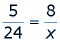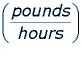Weight often affects cooking time.  Consider the following hypothetical situation: we are cooking an 8 pound turkey for Christmas dinner.  If the turkey needs to thaw in the refrigerator for 24 hours, per 5 pounds, we need to take the turkey out of the freezer in advance.  We can use a proportional relation to help us decide how early to thaw the turkey.The above proportion reads as follows:  5 pounds is to 24 hours as 8 pounds is to x hours.  By cross-multiplying and dividing, we can find an answer of 38.4 hours, which is the solution for x
If we are instructed to cook the turkey for 20 minutes per pound, how long do we need to cook the turkey?  Well, 20 minutes per pound for 8 pounds is 20 x 8 = 160 minutes.  And, 160 minutes is two hours and 40 minutes.  If we only knew the weight of our turkey in kilograms, we would need another conversion formula (kilograms to pounds) to find the weight of the turkey in pounds first, and then apply the recommendations.